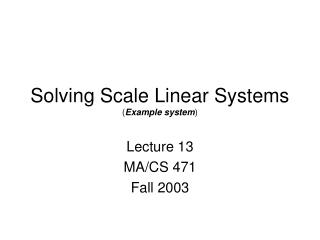DownloadDownload PresentationSolving Scale Linear Systems ( Example system )

# Solving Scale Linear Systems ( Example system )

Download Presentation## Solving Scale Linear Systems ( Example system )

- - - - - - - - - - - - - - - - - - - - - - - - - - - E N D - - - - - - - - - - - - - - - - - - - - - - - - - - -
##### Presentation Transcript

1. Solving Scale Linear Systems (Example system) Lecture 13 MA/CS 471 Fall 2003

2. First – Brief Reintroduction to Linear Systems • First we will use an example physical system to construct a set of 5 couple linear equations in 5 unknowns. • We will seek a solution using Matlab • Later we will consider generalizations to larger systems (with correspondingly more unknowns to find).

3. Circuit Problem 5W 1W 4W 6W 3W 7W + - 30V 1W 2W Problem: Find the current running through each closed loop

4. Circuit Problem 5W 1W 4W DC Battery Resistor (resistance in ohms) Resistance free wire 6W 3W 7W + - 30V 1W 2W Notation

5. Circuit Problem 5W 1W 4W 6W 3W 7W 30V 1W 2W Find the current (in amperes) traveling in the shown closed loops

6. Kirchoff’s Second Law • Kirchoff's 2nd Law states that for any closed loop path around a circuit the sum of the voltage gains and voltage drops equals zero. In the circuit shown, there is a voltage gain for each electron traveling through the voltage source and a voltage drop across the resistor.

7. Loop 1 Balance Consider LOOP 1 5W 1W 4W 6W I2 3W 7W I1 30V I3 1W 2W The gain is 30V. The loop 1 loss (by Ohm’s law) is: The gain due to current from loop 2 is: The gain due to current from loop 3 is: Kirchoff’s 2nd law states gain=loss, =>

8. All Loop Balances 5 5W 1W 4W 6W 3W 4 2 7W 30V 1 1W 2W 3

9. Rearranging Linear System Arranging unknown Loop currents on lefthand side and known voltage sources on right hand side: Divide through by Ohms:

10. Final System Simplifying the coefficients: Matrix form:

11. Final Form • Negating both sides: • This is the enemy. • We will create systems with a large number of degrees of freedom later on.

12. Solution (by Matlab) 1.08A 5W Solution: 1W 4W 6W 1.97A 2.74A 3W 8.19A 7W 5.46A 30V 1W 2W

13. Homework/Lab work Q1) • Create a non-trivial circuit with 15 sub loops. Use a range of resistor values between 1 and 10. • Using a sparse matrix (see MA375/Lecture 8 intro), solve for loop currents with Matlab • Draw a diagram indicating current along each segment of circuit (to two significant figures). • Verify Kerchoff’s first law (look it up) by checking the sum of currents at three of the wire intersections. • Count the number of non-zeros of your 15x15 matrix and report the amount of fill (i.e. number of non-zeros/225) • Include print out of matlab window used for matrix solution. Q2) Review: a) LU factorization b) condition number of a matrix